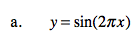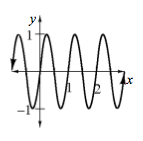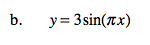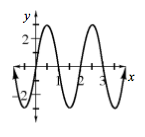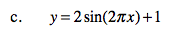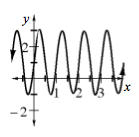Home > A2C > Chapter 8 > Lesson 8.2.3 > Problem8-145

8-145.
1. Sketch the graph of each equation below. Homework Help ✎

1. y = sin(2πx)

2. y = 3sin(πx)

3. y = 2sin(2πx) + 1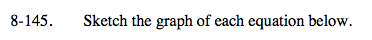For each of the following, consider the amplitude, period, horizontal shift, and vertical shift.